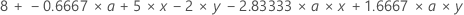# Calculate fitted values for general linear model (GLM)

## Scenario for examples

Suppose the design has 2 factors (Factor1 and Factor2). Factor1 has two levels (a and b) and Factor2 has three levels (x, y, and z). The data for Factor1 are in C1, Factor2 are in C2, and the responses are in C3. You perform General Linear Model with Factor1, Factor2, and the 2-way interaction Factor1*Factor2 in the model.

## Example of letting Minitab calculate the fitted values and store them in the worksheet

This option allows to you determine the fitted values using the values in the worksheet.

1. Choose Stat > ANOVA > General Linear Model > Fit General Linear Model.
2. In Responses, enter C3. In Factors, enter Factor1 Factor2.
3. Click Model. In the field under Factors and covariates select both 'Factor 1' and 'Factor 2'. Verify that 2 is selected in the field beside Interactions through order.
4. Click Add, then click OK.
5. Click Storage. Check Fits.
6. Click OK in each dialog box.

The fitted values are stored in the next available blank column in the worksheet, named FITS1.

## Example of entering coded values into the equation

Suppose you obtain the following coefficients in the output:

```Term                 Coef SE Coef      T      P
Constant           8.0000  0.5528  14.47  0.000
Factor1
a                 -0.6667  0.5528  -1.21  0.273
Factor2
x                  5.0000  0.7817   6.40  0.001
y                 -2.0000  0.7817  -2.56  0.043
Factor1*Factor2
a       x         -2.8333  0.7817  -3.62  0.011
a       y          1.6667  0.7817   2.13  0.077```
1. Using the coefficients from the table above, you can obtain the following regression equation.

The equation is:Using the default coding that Minitab uses:
• If Factor1 is a, use a = 1
• If Factor1 is b, use a = –1
• If Factor2 is x, use x = 1 and y = 0
• If Factor2 is y, use x = 0 and y = 1
• If Factor2 is z, use x = –1 and y = –1
2. Put the factor levels in to the equation.

Suppose the 9th row in the data set has Factor1 = b and Factor2 = z. The fitted value is:

= 8.0000 + - 0.6667*-1 + 5.0000*-1 - 2.0000*-1 - 2.8333*-1*-1 + 1.6667*-1*-1

= 8.0000 + 0.6667 - 5.0000 + 2.0000 - 2.8333 + 1.6667

= 4.5

If you choose to store the fits as described in Option 1, you will see 4.5 in row 9 (with Factor1 = b and Factor2 = z) of the FITS1 column.

## How to display all the coefficients

You can have Minitab display the coefficients that are not displayed by default.

1. Choose Stat > ANOVA > General Linear Model > Fit General Linear Model.
2. Enter the response columns and factor columns.
3. Click Results and beside Coefficients select Full set of coefficients.
4. Click OK in each dialog box.
By using this site you agree to the use of cookies for analytics and personalized content.  Read our policy